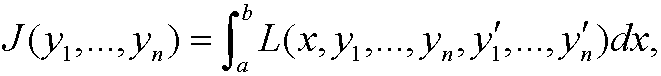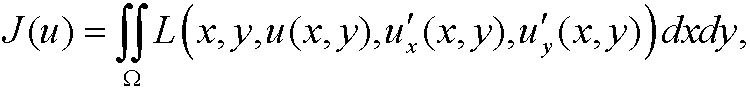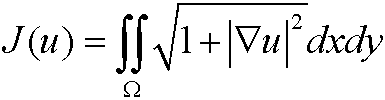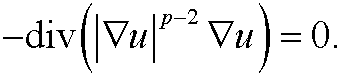# 10. The Euler equation for some more general cases.

1. Higher derivatives: Assume that we have a functional of the typewhere y  is a function in C2n[a,b] that fulfills the boundary conditionsThe Euler equationen is then2. Several functions: Assume that we have a functional of the typewhere yi, i=1,…,n  are functions in C2[a,b] that fulfill the boundary conditions<>The Euler equation is then

<>3. Several variables: Assume that we have a functional of the typewhere u  is a function in C2()  that fulfills the boundary conditionThe Euler equation is thenExample 14: (Compare example 9.) The Euler equationen tois Laplace’ equationExample 15: (Compare example 8.) The Euler equation tois the nonlinear differential equationExample 16: The Euler equation to the functionalis p-Laplace equationThis equation is also called the p-harmonic equation and is sometimes used to model non-Newtonian flows.

www.larserikpersson.se